Elementary Algebra 2e

# 9.7Higher Roots

Elementary Algebra 2e9.7 Higher Roots

### Learning Objectives

By the end of this section, you will be able to:

• Simplify expressions with higher roots
• Use the Product Property to simplify expressions with higher roots
• Use the Quotient Property to simplify expressions with higher roots
• Add and subtract higher roots

### Be Prepared 9.18

Before you get started, take this readiness quiz.

Simplify: $y5y4y5y4$.
If you missed this problem, review Example 6.18.

### Be Prepared 9.19

Simplify: $(n2)6(n2)6$.
If you missed this problem, review Example 6.22.

### Be Prepared 9.20

Simplify: $x8x3x8x3$.
If you missed this problem, review Example 6.59.

### Simplify Expressions with Higher Roots

Up to now, in this chapter we have worked with squares and square roots. We will now extend our work to include higher powers and higher roots.

Let’s review some vocabulary first.

$We write:We say:n2nsquaredn3ncubedn4nto the fourthn5nto the fifthWe write:We say:n2nsquaredn3ncubedn4nto the fourthn5nto the fifth$

The terms ‘squared’ and ‘cubed’ come from the formulas for area of a square and volume of a cube.

It will be helpful to have a table of the powers of the integers from $−5to5−5to5$. See Figure 9.4.

Figure 9.4 First through fifth powers of integers from $−5−5$ to $5.5.$

Notice the signs in Figure 9.4. All powers of positive numbers are positive, of course. But when we have a negative number, the even powers are positive and the odd powers are negative. We’ll copy the row with the powers of $−2−2$ below to help you see this.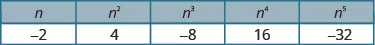Earlier in this chapter we defined the square root of a number.

$Ifn2=m,thennis a square root ofm.Ifn2=m,thennis a square root ofm.$

And we have used the notation $mm$ to denote the principal square root. So $m≥0m≥0$ always.

We will now extend the definition to higher roots.

### nth Root of a Number

If $bn=abn=a$, then $bb$ is an nth root of a number $aa$.

The principal nth root of $aa$ is written $anan$.

n is called the index of the radical.

We do not write the index for a square root. Just like we use the word ‘cubed’ for $b3b3$, we use the term ‘cube root’ for $a3a3$.

We refer to Figure 9.4 to help us find higher roots.

$43=64643=434=81814=3(−2)5=−32−325=−243=64643=434=81814=3(−2)5=−32−325=−2$

Could we have an even root of a negative number? No. We know that the square root of a negative number is not a real number. The same is true for any even root. Even roots of negative numbers are not real numbers. Odd roots of negative numbers are real numbers.

### Properties of $a n a n$

When $nn$ is an even number and

• $a≥0a≥0$, then $anan$ is a real number
• $a<0a<0$, then $anan$ is not a real number

When $nn$ is an odd number, $anan$ is a real number for all values of $aa$.

### Example 9.88

Simplify: $8383$ $814814$ $325325$.

### Try It 9.175

Simplify: $273273$ $25642564$ $24352435$.

### Try It 9.176

Simplify: $1000310003$ $164164$ $325325$.

### Example 9.89

Simplify: $−643−643$ $−164−164$ $−2435−2435$.

### Try It 9.177

Simplify: $−1253−1253$ $−164−164$ $−325−325$.

### Try It 9.178

Simplify: $−2163−2163$ $−814−814$ $−10245−10245$.

When we worked with square roots that had variables in the radicand, we restricted the variables to non-negative values. Now we will remove this restriction.

The odd root of a number can be either positive or negative. We have seen that $−643=−4−643=−4$.

But the even root of a non-negative number is always non-negative, because we take the principal nth root.

Suppose we start with $a=−5a=−5$.

$(−5)4=6256254=5(−5)4=6256254=5$

How can we make sure the fourth root of −5 raised to the fourth power, $(−5)4(−5)4$ is 5? We will see in the following property.

### Simplifying Odd and Even Roots

For any integer $n≥2n≥2$,

$whennis oddann=awhennis evenann=|a|whennis oddann=awhennis evenann=|a|$

We must use the absolute value signs when we take an even root of an expression with a variable in the radical.

### Example 9.90

Simplify: $x2x2$ $n33n33$ $p44p44$ $y55y55$.

### Try It 9.179

Simplify: $b2b2$ $w33w33$ $m44m44$ $q55q55$.

### Try It 9.180

Simplify: $y2y2$ $p33p33$ $z44z44$ $q55q55$.

### Example 9.91

Simplify: $y183y183$ $z84z84$.

### Try It 9.181

Simplify: $u124u124$ $v153v153$.

### Try It 9.182

Simplify: $c205c205$ $d246d246$.

### Example 9.92

Simplify: $64p6364p63$ $16q12416q124$.

### Try It 9.183

Simplify: $27x27327x273$ $81q28481q284$.

### Try It 9.184

Simplify: $125p93125p93$ $243q255243q255$.

### Use the Product Property to Simplify Expressions with Higher Roots

We will simplify expressions with higher roots in much the same way as we simplified expressions with square roots. An nth root is considered simplified if it has no factors of $mnmn$.

### Simplified nth Root

$anan$ is considered simplified if $aa$ has no factors of $mnmn$.

We will generalize the Product Property of Square Roots to include any integer root $n≥2n≥2$.

### Product Property of nth Roots

$abn=an·bnandan·bn=abnabn=an·bnandan·bn=abn$

when $anan$ and $bnbn$ are real numbers and for any integer $n≥2n≥2$

### Example 9.93

Simplify: $x43x43$ $x74x74$.

### Try It 9.185

Simplify: $y64y64$ $z53z53$.

### Try It 9.186

Simplify: $p85p85$ $q136q136$.

### Example 9.94

Simplify: $163163$ $24342434$.

### Try It 9.187

Simplify: $813813$ $644644$.

### Try It 9.188

Simplify: $62536253$ $72947294$.

Don’t forget to use the absolute value signs when taking an even root of an expression with a variable in the radical.

### Example 9.95

Simplify: $24x7324x73$ $80y14480y144$.

### Try It 9.189

Simplify: $54p10354p103$ $64q10464q104$.

### Try It 9.190

Simplify: $128m113128m113$ $162n74162n74$.

### Example 9.96

Simplify: $−273−273$ $−164−164$.

### Try It 9.191

Simplify: $−1083−1083$ $−484−484$.

### Try It 9.192

Simplify: $−6253−6253$ $−3244−3244$.

### Use the Quotient Property to Simplify Expressions with Higher Roots

We can simplify higher roots with quotients in the same way we simplified square roots. First we simplify any fractions inside the radical.

### Example 9.97

Simplify: $a8a53a8a53$ $a10a24a10a24$.

### Try It 9.193

Simplify: $x7x34x7x34$ $y17y54y17y54$.

### Try It 9.194

Simplify: $m13m73m13m73$ $n12n25n12n25$.

Previously, we used the Quotient Property ‘in reverse’ to simplify square roots. Now we will generalize the formula to include higher roots.

### Quotient Property of nth Roots

$abn=anbnandanbn=abnabn=anbnandanbn=abn$

when $anandbnare real numbers,b≠0,and for any integern≥2anandbnare real numbers,b≠0,and for any integern≥2$

### Example 9.98

Simplify: $−108323−108323$ $96x743x2496x743x24$.

### Try It 9.195

Simplify: $−532323−532323$ $486m1143m54486m1143m54$.

### Try It 9.196

Simplify: $−192333−192333$ $324n742n34324n742n34$.

If the fraction inside the radical cannot be simplified, we use the first form of the Quotient Property to rewrite the expression as the quotient of two radicals.

### Example 9.99

Simplify: $24x7y3324x7y33$ $48x10y8448x10y84$.

### Try It 9.197

Simplify: $108c10d63108c10d63$ $80x10y5480x10y54$.

### Try It 9.198

Simplify: $40r3s340r3s3$ $162m14n124162m14n124$.

### Add and Subtract Higher Roots

We can add and subtract higher roots like we added and subtracted square roots. First we provide a formal definition of like radicals.

• $942x4942x4$ and $−242x4−242x4$ are like radicals.
• $5125x35125x3$ and $6125y36125y3$ are not like radicals. The radicands are different.
• $21000q521000q5$ and $−41000q4−41000q4$ are not like radicals. The indices are different.

We add and subtract like radicals in the same way we add and subtract like terms. We can add $942x4+(−242x4)942x4+(−242x4)$ and the result is $742x4742x4$.

### Example 9.100

Simplify: $4x3+4x34x3+4x3$ $484−284484−284$.

### Try It 9.199

Simplify: $3x5+3x53x5+3x5$ $393−93393−93$.

### Try It 9.200

Simplify: $10y4+10y410y4+10y4$ $5326−33265326−3326$.

When an expression does not appear to have like radicals, we will simplify each radical first. Sometimes this leads to an expression with like radicals.

### Example 9.101

Simplify: $543−163543−163$ $484+2434484+2434$.

### Try It 9.201

Simplify: $1923−8131923−813$ $324+5124324+5124$.

### Try It 9.202

Simplify: $1283−25031283−2503$ $645+4865645+4865$.

### Example 9.102

Simplify: $24x43−−81x7324x43−−81x73$ $162y94+512y54162y94+512y54$.

### Try It 9.203

Simplify: $32y53−−108y8332y53−−108y83$ $243r114+768r104243r114+768r104$.

### Try It 9.204

Simplify: $40z73−−135z4340z73−−135z43$ $80s134+1280s6480s134+1280s64$.

### Media

Access these online resources for additional instruction and practice with simplifying higher roots.

### Section 9.7 Exercises

#### Practice Makes Perfect

Simplify Expressions with Higher Roots

In the following exercises, simplify.

442.

$21632163$ $25642564$ $325325$

443.

$273273$ $164164$ $24352435$

444.

$51235123$ $814814$ $1515$

445.

$12531253$ $1296412964$ $1024510245$

446.

$−83−83$ $−814−814$ $−325−325$

447.

$−643−643$ $−164−164$ $−2435−2435$

448.

$−1253−1253$ $−12964−12964$ $−10245−10245$

449.

$−5123−5123$ $−814−814$ $−15−15$

450.

$u55u55$ $v88v88$

451.
1. $a33a33$

2.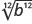452.

$y44y44$ $m77m77$

453.

$k88k88$ $p66p66$

454.

$x93x93$ $y124y124$

455.

$a105a105$ $b273b273$

456.

$m84m84$ $n205n205$

457.

$r126r126$ $s303s303$

458.

$16x8416x84$ $64y12664y126$

459.

$−8c93−8c93$ $125d153125d153$

460.

$216a63216a63$ $32b20532b205$

461.

$128r147128r147$ $81s24481s244$

Use the Product Property to Simplify Expressions with Higher Roots

In the following exercises, simplify.

462.

$r53r53$ $s104s104$

463.

$u75u75$ $v116v116$

464.

$m54m54$ $n108n108$

465.

$p85p85$ $q83q83$

466.

$324324$ $647647$

467.

$62536253$ $12861286$

468.

$645645$ $25632563$

469.

$3125431254$ $813813$

470.

$108x53108x53$ $48y6448y64$

471.

$96a7596a75$ $375b43375b43$

472.

$405m104405m104$ $160n85160n85$

473.

$512p53512p53$ $324q74324q74$

474.

$−8643−8643$ $−2564−2564$

475.

$−4865−4865$ $−646−646$

476.

$−325−325$ $−18−18$

477.

$−83−83$ $−164−164$

Use the Quotient Property to Simplify Expressions with Higher Roots

In the following exercises, simplify.

478.

$p11p23p11p23$ $q17q134q17q134$

479.

$d12d75d12d75$ $m12m48m12m48$

480.

$u21u115u21u115$ $v30v126v30v126$

481.

$r14r53r14r53$ $c21c94c21c94$

482.

$6442464424$ $128x852x25128x852x25$

483.

$−625353−625353$ $80m745m480m745m4$

484.

$125023125023$ $486y92y34486y92y34$

485.

$1626316263$ $160r105r34160r105r34$

486.

$54a8b3354a8b33$ $64c5d2464c5d24$

487.

$96r11s3596r11s35$ $128u7v36128u7v36$

488.

$81s8t3381s8t33$ $64p15q12464p15q124$

489.

$625u10v33625u10v33$ $729c21d84729c21d84$

In the following exercises, simplify.

490.

$8p7+8p78p7+8p7$ $3253−2533253−253$

491.

$15q3+15q315q3+15q3$ $2274−62742274−6274$

492.

$39x5+79x539x5+79x5$ $83q7−23q783q7−23q7$

493.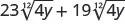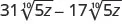494.

$813−1923813−1923$ $5124−3245124−324$

495.

$2503−5432503−543$ $2434−187542434−18754$

496.

$1283+25031283+2503$ $7295+9657295+965$

497.

$2434+125042434+12504$ $20003+54320003+543$

498.

$64a103−−216a12364a103−−216a123$ $486u74+768u34486u74+768u34$

499.

$80b53−−270b3380b53−−270b33$ $160v104−1280v34160v104−1280v34$

Mixed Practice

In the following exercises, simplify.

500.

$16 4 16 4$

501.

$64 6 64 6$

502.

$a 3 3 a 3 3$

503.504.

$−8 c 9 3 −8 c 9 3$

505.

$125 d 15 3 125 d 15 3$

506.

$r 5 3 r 5 3$

507.

$s 10 4 s 10 4$

508.

$108 x 5 3 108 x 5 3$

509.

$48 y 6 4 48 y 6 4$

510.

$−486 5 −486 5$

511.

$−64 6 −64 6$

512.

$64 4 2 4 64 4 2 4$

513.

$128 x 8 5 2 x 2 5 128 x 8 5 2 x 2 5$

514.

$96 r 11 s 3 5 96 r 11 s 3 5$

515.

$128 u 7 v 3 6 128 u 7 v 3 6$

516.

$81 3 − 192 3 81 3 − 192 3$

517.

$512 4 − 32 4 512 4 − 32 4$

518.

$64 a 10 3 − −216 a 12 3 64 a 10 3 − −216 a 12 3$

519.

$486 u 7 4 + 768 u 3 4 486 u 7 4 + 768 u 3 4$

#### Everyday Math

520.

Population growth The expression $10·xn10·xn$ models the growth of a mold population after $nn$ generations. There were 10 spores at the start, and each had $xx$ offspring. So $10·x510·x5$ is the number of offspring at the fifth generation. At the fifth generation there were 10,240 offspring. Simplify the expression $10,24010510,240105$ to determine the number of offspring of each spore.

521.

Spread of a virus The expression $3·xn3·xn$ models the spread of a virus after $nn$ cycles. There were three people originally infected with the virus, and each of them infected $xx$ people. So $3·x43·x4$ is the number of people infected on the fourth cycle. At the fourth cycle 1875 people were infected. Simplify the expression $187534187534$ to determine the number of people each person infected.

#### Writing Exercises

522.

Explain how you know that $x105=x2x105=x2$ .

523.

Explain why $−644−644$ is not a real number but $−643−643$ is.

#### Self Check

After completing the exercises, use this checklist to evaluate your mastery of the objectives of this section.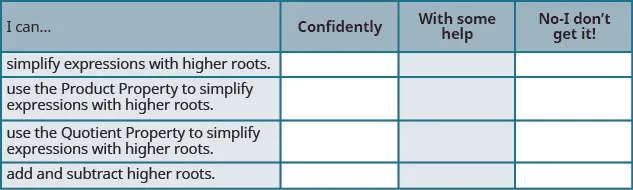What does this checklist tell you about your mastery of this section? What steps will you take to improve?

Order a print copy

As an Amazon Associate we earn from qualifying purchases.